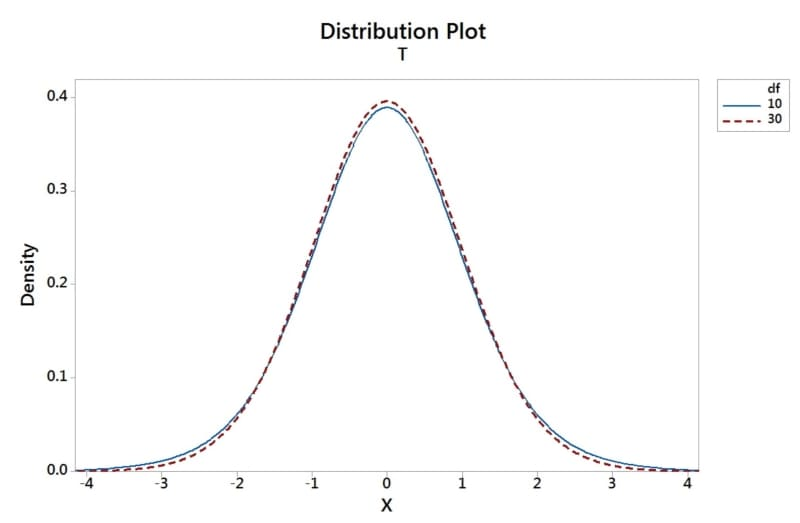Home / Blog / Data Science / Z Table Statistics and Chart

# Z Table Statistics and Chart

• July 08, 2023
• 25229
• 326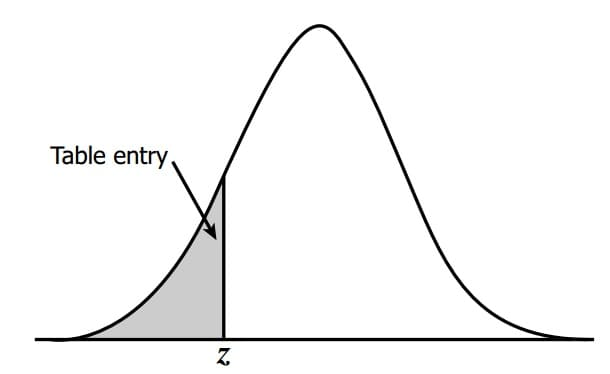### Meet the Author : Mr. Sharat Chandra

Sharat Chandra is the head of analytics at 360DigiTMG as well as one of the founders and directors of Innodatatics Private Limited. With more than 17 years of work experience in the IT sector and Worked as a Data scientist for 14+ years across several industry domains, Sharat Chandra has a wide range of expertise in areas like retail, manufacturing, medical care, etc. With over ten years of expertise as the head trainer at 360DigiTMG, Sharat Chandra has been assisting his pupils in making the move to the IT industry simple. Along with the Oncology team, he made a contribution to the field of LSHC, especially to the field of cancer therapy, which was published in the British magazine of Cancer research magazine.

## Z Table

Standard is represented by Z variable. Random variable that is normal and has a mean of 0 and standard deviation of 1

The area under the curve, which totals 1, is shown in the Z table. According to six sigma ideas, the Z tables are really computed over a range of +/- 3.4 values.

### Shows the percent of population:

• Less than or equal to Z (option "Up to Z")
• Greater than Z (option "Z onward") is calculated as 1 - (less than or equal to Z)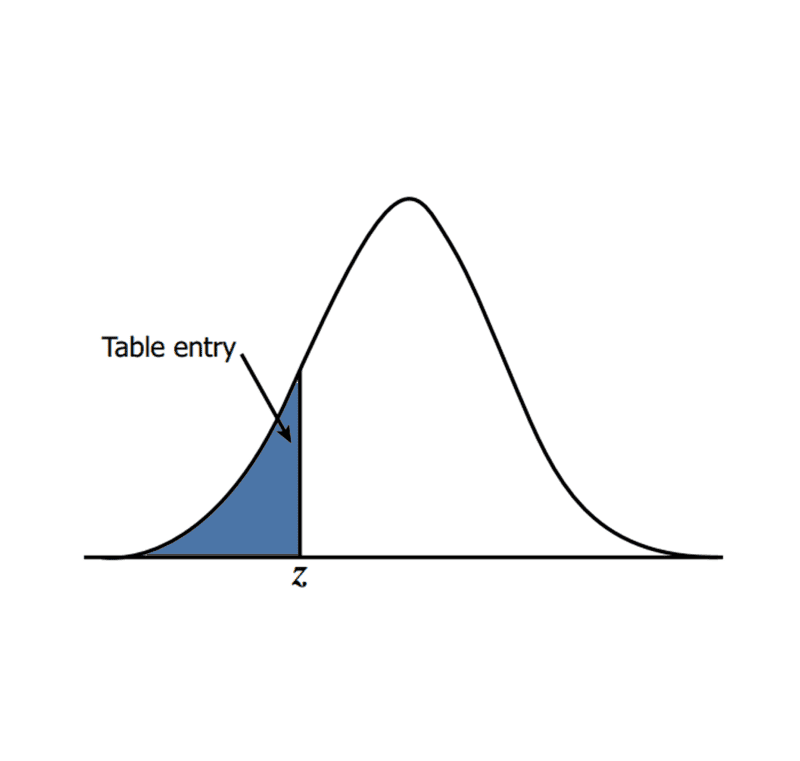### Example 1:Learn how to calculate a Continuous Random Variable's probability using the Z table.

Assume that a continuous random variable representing students' test results has a normal distribution with the values = 711 and = 29.

What is the likelihood that pupils will receive a score of x > 680?

Ans: Standard normal variable, z value calculated for corresponding continuous random variable x (680)

Step 1: Calculate z score corresponding to 680

formula: z = (x - µ) / σ

z = (680-711)/29

z = -1.06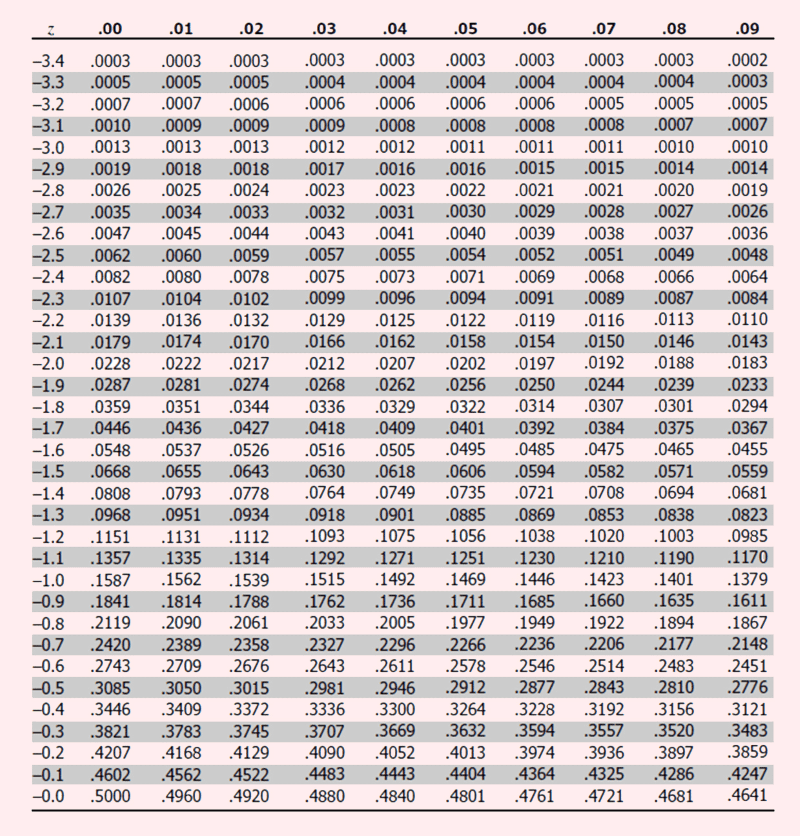Step 2: Refer to z table:

Utilise the z table to calculate the probability.

Start reading the second decimal number along column 06 at the row where z = -1.0.

The value is 0.1446 in the z table with a z value of -1.06.

This suggests that the area under the curve from -1.06 of z value (towards the left tail) is around 14.46%.

This may be viewed as having a probability of 14.46% for the population of students with scores between 0 and 680 for the supplied normal distributed data.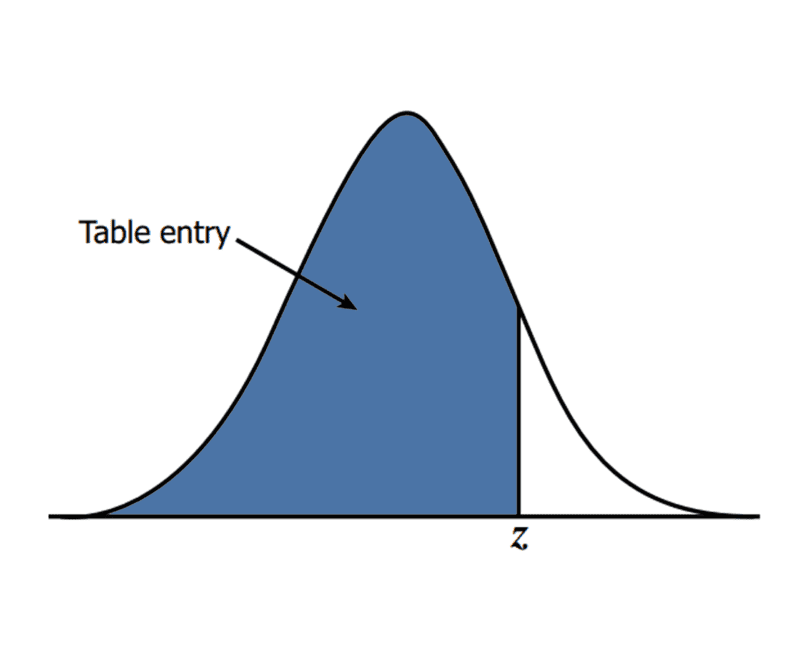Example 2: Assume a continuous random variable students examination scores following a normal distribution, with µ = 711, σ = 29

Find out the probability of students securing a score of x > 725?

Ans: Standard normal variable, z value calculated for corresponding continuous random variable x (725)

Step 1: Calculate z score corresponding to 725

formula: z = (x - µ) / σ

z = (725 - 711)/29

z = 0.48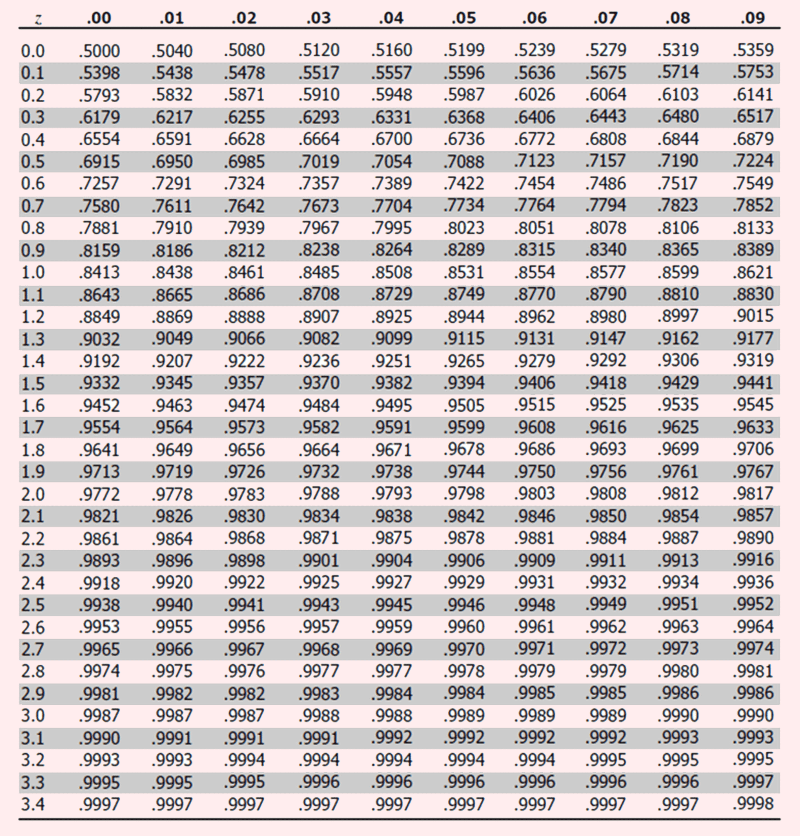Step 2:

Utilise the z table to calculate the probability.

Start reading along column 08. The second decimal value is found starting at the row with z = 0.4.

The value is 0.6844 in the z table when the z value is 0.48.

This suggests that the area under the curve from z=0.48 towards the left tail is around 68.44%.

This may be translated as having a chance of 68.44% for the population of students whose scores lie between 0 and 725.

The area under the curve (probability), from a particular quartile value (z value), towards the left tail of the curve, is represented by the table, commonly known as the Standard Normal Table.

### Data Analyst Courses in Other Locations